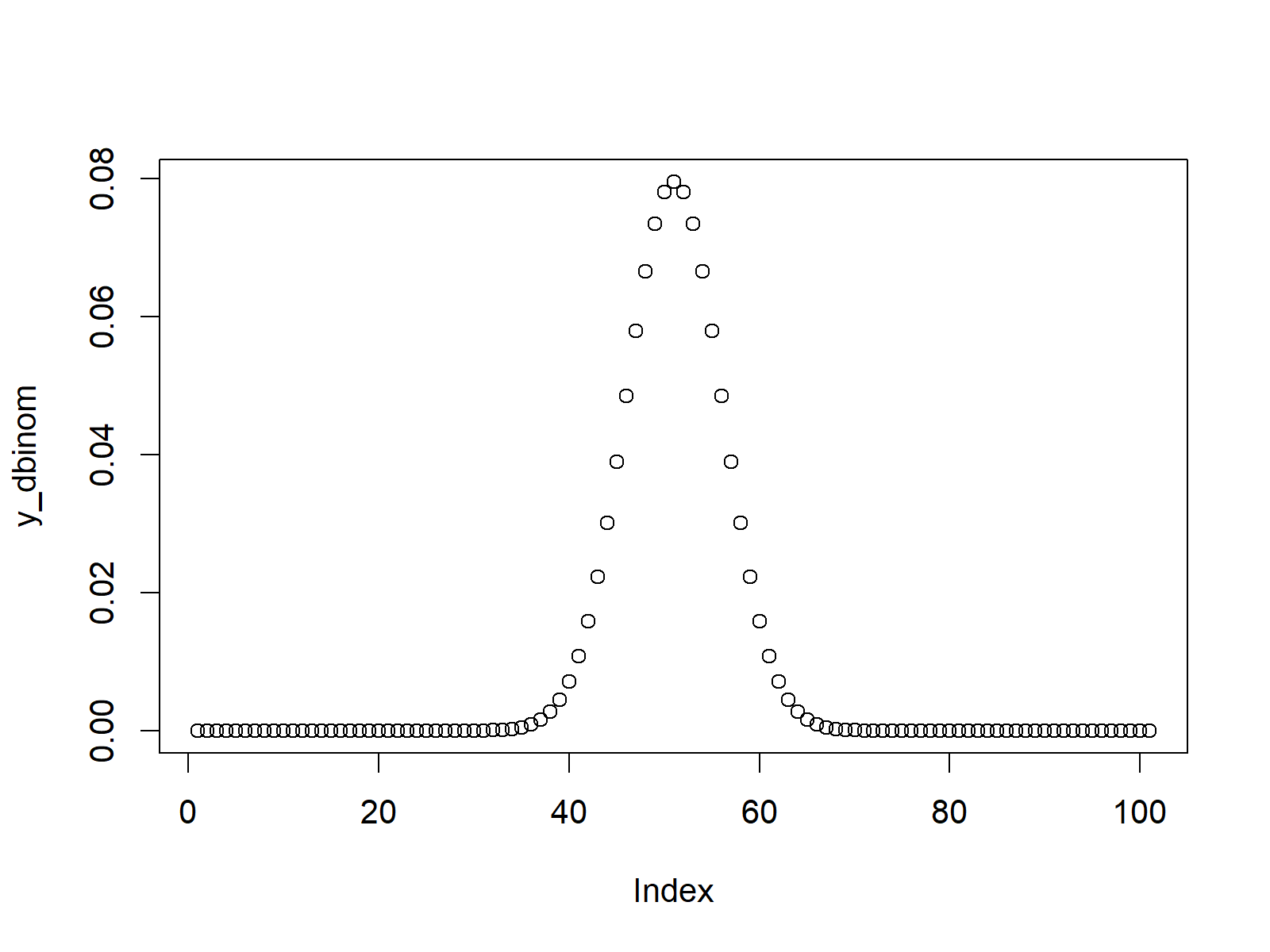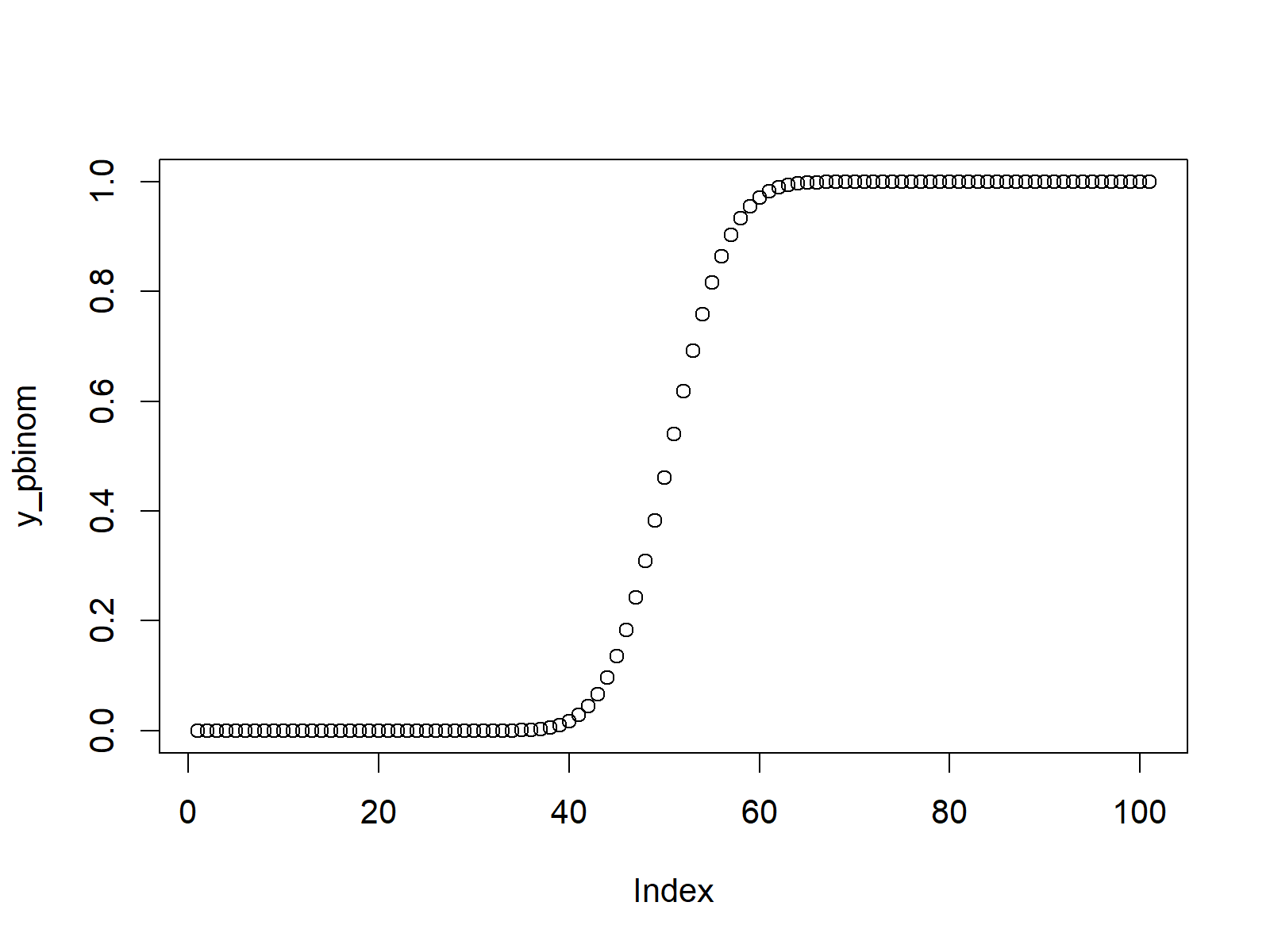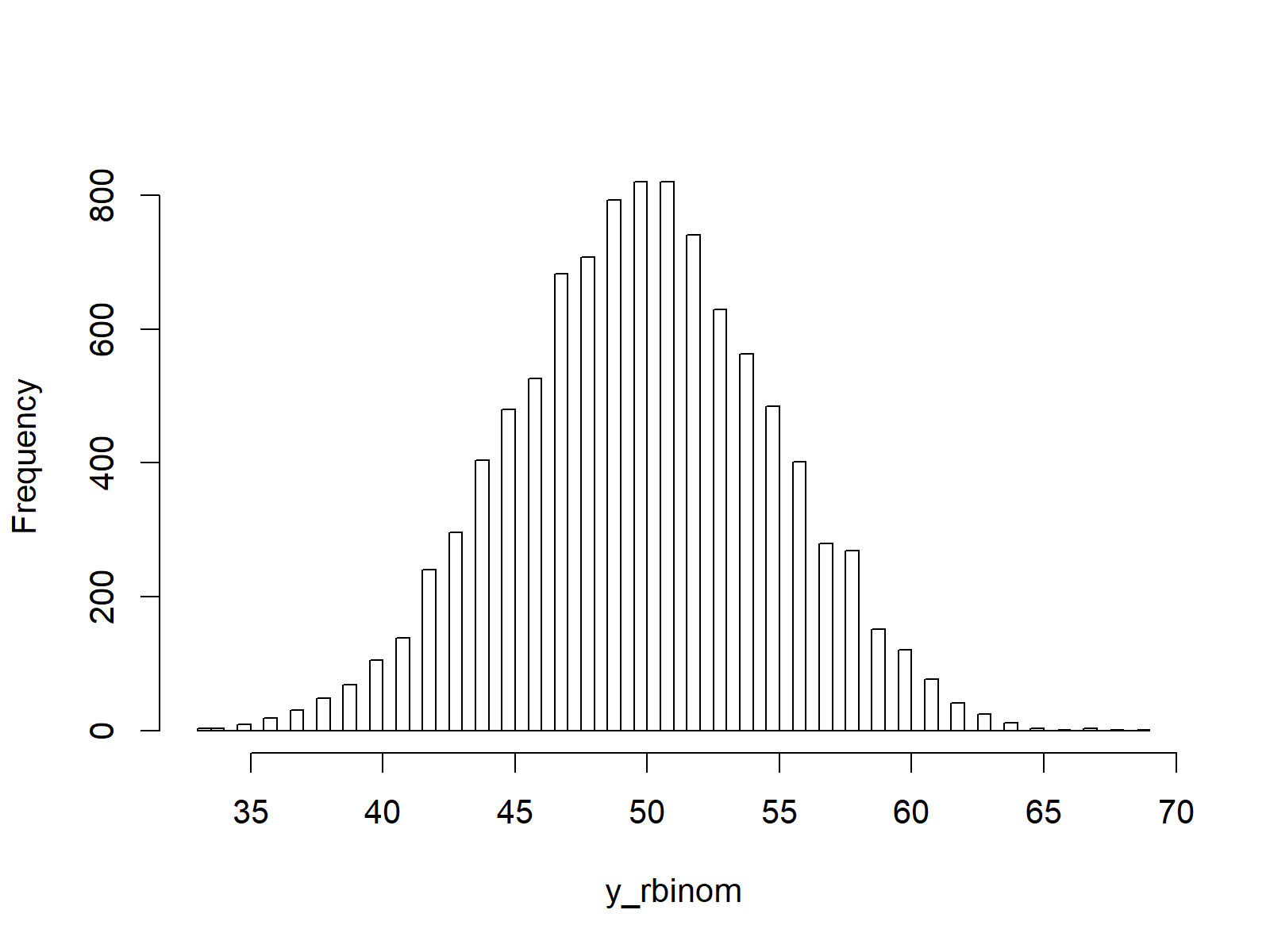# Binomial Distribution in R (4 Examples) | dbinom, pbinom, qbinom & rbinom Functions

In this tutorial you’ll learn how to apply the binom functions in R programming.

The tutorial is structured as follows:

Let’s take a look at some R codes in action!

## Example 1: Binomial Density in R (dbinom Function)

In the first example, we’ll create an R plot of the binomial density.

First, we have to create a vector of quantiles as input for the dbinom R function:

`x_dbinom <- seq(0, 100, by = 1)                       # Specify x-values for binom function`

Then, we can apply the dbinom function to this vector as shown below. Note that I have specified the size to be equal to 100 (i.e. the number of trials) and the probability for each binomial draw to be equal to 0.5 (i.e. 50%). Of cause you can modify these arguments as you want.

`y_dbinom <- dbinom(x_dbinom, size = 100, prob = 0.5)  # Apply dbinom function`

If we want to illustrate the output of the dbinom function in a graphic, we can use the plot function:

`plot(y_dbinom)                                        # Plot dbinom values`Figure 1: Binomially Distributed Density.

Figure 1 shows the output of the previous R code – A binomially distributed density.

## Example 2: Binomial Cumulative Distribution Function (pbinom Function)

In Example 2, I’ll explain how to apply the pbinom function to create a plot of the binomial cumulative distribution function (CDF) in R. First, we need to create an input vector (as in Example 1).

`x_pbinom <- seq(0, 100, by = 1)                       # Specify x-values for pbinom function`

Now, we can apply the pbinom command…

`y_pbinom <- pbinom(x_pbinom, size = 100, prob = 0.5)  # Apply pbinom function`

…and draw a plot of the binomial CDF:

`plot(y_pbinom)                                        # Plot pbinom values`Figure 2: Binomial CDF in R.

## Example 3: Binomial Quantile Function (qbinom Function)

In this example, you’ll learn how to plot the binomial quantile function in R. As a first step, we have to create a sequence of probabilities:

`x_qbinom <- seq(0, 1, by = 0.01)                      # Specify x-values for qbinom function`

Then, we can apply the qbinom function to get the corresponding value of the binomial quantile function for each value in our sequence of probabilities:

`y_qbinom <- qbinom(x_qbinom, size = 100, prob = 0.5)  # Apply qbinom function`

Finally, we can illustrate the output in an R plot:

`plot(y_qbinom)                                        # Plot qbinom values`Figure 3: Quantile Function of Binomial Distribution.

## Example 4: Simulation of Random Numbers (rbinom Function)

If we want to generate some random numbers with a binomial distribution in R, we can use the rbinom function. Let’s specify a seed for reproducibility…

`set.seed(13579)                                       # Set seed for reproducibility`

…and a sample size of random numbers that we want to draw:

`N <- 10000                                            # Specify sample size`

Now, we can create a set of random numbers with a binomial distribution based on the rbinom function:

```y_rbinom <- rbinom(N, size = 100, prob = 0.5)         # Draw N binomially distributed values
y_rbinom                                              # Print values to RStudio console
# 45 44 55 43 35 47 56 52 49 51 47 50 51 54 53 48 57 55 51...```

The RStudio console shows the random numbers that we have just created. They have a (theoretical) range between 0 and 100 (i.e. 0 positive outcomes or 100 positive outcomes).

We can illustrate the distribution of our random numbers in a histogram:

```hist(y_rbinom,                                        # Plot of randomly drawn binomial density
breaks = 100,
main = "")```Figure 4: Random Numbers Generated According to Binomial Distribution.

Note that in the previous R syntax we used a size of 100 trials and a probability of success of 0.5. In case we want to generate a random dummy variable, we simply have to set the size argument to be equal to 1:

```y_rbinom_dummy <- rbinom(N, size = 1, prob = 0.5)     # Draw N dummy values
y_rbinom_dummy                                        # Print values to RStudio console
# 0 1 0 1 1 1 0 1 0 0 0 0 1 0 1 0 0 1 1 1 1 1 0 0 0 0 0 1 1 1 1 0 1 1 1...```

## Video, Further Resources & Summary

If you need more information on the topics of this article, you may want to have a look at the following video of my YouTube channel. I show the R programming code of this tutorial in the video:

You could also have a look at the other posts on distributions and the generation of random numbers in R:

In addition, you could have a look at the related tutorials on this homepage. I have published numerous related posts already.

By the way, if you are looking for a job where the skills learned in this tutorial can be applied, you may have a look at the R programming & data science job offers on Jooble. Jooble is an ad partner of Statistics Globe and displays job offers from thousands of different sources.

In summary: In this tutorial you learned how to use the binom functions in R. Let me know in the comments below, if you have additional questions.

Subscribe to the Statistics Globe Newsletter